Processing ......FreeComputerBooks.com Links to Free Computer, Mathematics, Technical Books all over the World

Mathematical/Computational Software, Mathematica, Sage, MATLAB, SAS®, etc.
Related Book Categories:
 MATLAB Programming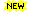Computational and Algorithmic Mathematics Algorithms and Data Structures Algebra and Abstract Algebra
•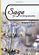Sage for Undergraduates (Gregory V. Bard)

This book is a gentle introduction to SageMath for undergraduate students toward the end of Calculus or higher-level course work such as Multivariate Calculus, Differential Equations, Linear Algebra, or Math Modeling.

•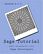Sage Tutorial (William Stein)

This tutorial is the best way to become familiar with SageMath in only a few hours. It explains how to use the free and open source mathematical software package Sage. Both binaries and source code are available for SageMath from the download page.

•Mathematica® Programming: an Advanced Introduction

Starting from first principles, this book covers all of the foundational material needed to develop a clear understanding of the Mathematica® language, with a practical emphasis on solving problems.

•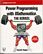Power Programming with Mathematica® (David B. Wagner)

This book unpacks Mathematica® for programmers, building insights into programming style via real world syntax, real world examples, and extensive parallels to other languages.

•Solving PDEs in Python: The FEniCS Tutorial I (H. Langtangen)

This book offers a concise and gentle introduction to finite element programming in Python based on the popular FEniCS software library. Using a series of examples, it guides readers through the essential steps to quickly solving a PDE in FEniCS.

•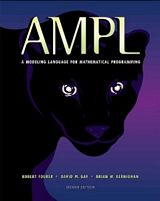AMPL: A Modeling Language for Mathematical Programming

This book is a complete guide to AMPL for modelers at all levels of experience. It begins with a tutorial on widely used linear programming models, and presents all of AMPL's features for linear programming with extensive examples.

•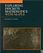Exploring Discrete Mathematics With Maple (Kenneth H. Rosen)

This is is a supplement in discrete mathematics to concentrate on the computational aspects of the computer algebra system Maple. Detailed instructions for the use of Maple are included in an introductory chapter and in each subsequent chapter.

•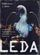LEDA: A Platform for Combinatorial and Geometric Computing

LEDA is a library of efficient data types and algorithms and a platform for combinatorial and geometric computing. This book, written by the main authors of LEDA, is the definitive account of how the system operates and how it can be used.

•Certified Programming with Dependent Types on Sat Nov 12, 2016

This book provides an introduction to the Coq software for writing and checking mathematical proofs. It takes a practical engineering focus throughout, emphasizing techniques that will help users to build, understand, and maintain large Coq code changes.

•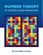Number Theory: In Context and Interactive, using SageMath

This is a textbook for an upper-level number theory course, with a clear vision to expose students to the connections to all areas of mathematics, and nearly every concept can be visualized or experimented with using the mathematics software SageMath.

•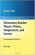Elementary Number Theory using SageMath (William Stein)

This is a book about prime numbers, congruences, secret messages, and elliptic curves that you can read cover to cover. Many numerical examples are given throughout the book using the SageMath mathematical software.

•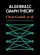Explorations in Algebraic Graph Theory with SageMath

This book aims to express properties of graphs in algebraic terms, then to deduce theorems about them. It tackles the applications of linear algebra and matrix theory to the study of graphs; algebraic constructions such as adjacency matrix, using the SageMath.

•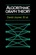Algorithmic Graph Theory using SageMath (David Joyner, et al)

This is an introductory book on algorithmic graph theory. Theory and algorithms are illustrated using the Sage open source mathematics software. It's especially suitable for computer scientists and mathematicians interested in computational complexity.

•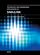Technology and Engineering Applications of SIMULINK

The subject matter of this book is to present the procedural steps required for modeling and simulating the basic dynamic system problems in SIMULINK (a supplementary part of MATLAB)

•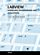Modeling, Programming and Simulations Using LabVIEW™

This book goes from simple or distributed control software to modeling done in LabVIEW to very specific applications to usage in the educational environment. It enables programming by implementing directly the equivalent of an SADT "actigram".

•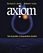Axiom: The Scientific Computation System (Richard D. Jenks, et al)

The book gives the reader a technical introduction to AXIOM, interacts with the system's tutorial, accesses algorithms newly developed by the symbolic computation community, and presents advanced programming and problem solving techniques.

•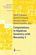Computations in Algebraic Geometry with Macaulay 2

This book presents algorithmic tools for algebraic geometry and experimental applications of them. Macaulay 2 is a computer algebra system devoted to supporting research in algebraic geometry, commutative algebra, and their applications.

•LAPACK95 Users' Guide (Vincent Barker, et al.)

This book provides an introduction to the design of the LAPACK95 package, a detailed description of its contents, reference manuals for the leading comments of the routines, and example programs.

•LAPACK Users' Guide, 3rd Edition (E. Anderson, Z. Bai, et al)

This free book provides an introduction to the design of the LAPACK package, a detailed description of its contents, reference manuals for the leading comments of the routines, and example programs

•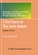A First Course on Time Series Analysis with SAS (Michael Falk, et al)

A unique feature of this book is its integration with the statistical software package SAS® (Statistical Analysis System) computing environment. Basic applied statistics is assumed through multiple regression.

•Step-By-Step Programming With Base SAS® Software

This book provides conceptual information about SAS® software along with step-by-step examples that illustrate the concepts. It answers every question that a new or intermediate SAS® user might have. Examples are thorough, readable, and clear.

•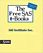Free SAS® e-Books (SAS Institute, Inc.)

Gain insight on SAS® solutions and analytics technology with a collection of free e-books, includes Artificial Intelligence, Machine Learning, Computer Vision, Natural Language Processing, Programming for R Users, etc.

•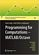Programming for Computations - MATLAB/Octave (Svein Linge)

This book presents computer programming as a key method for solving mathematical problems using MATLAB and Octave. It is intended for novice programmers and engineers. Each treated concept is illustrated and explained in detail by means of working examples.

•Scientific Computing with MATLAB (Paul Gribble)

The goal of this book is to provide you with skills in scientific computing: tools and techniques that you can use in your own scientific research. We will focus on learning to think about experiments and data in a computational framework.

•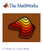Experiments with MATLAB (Cleve Moler)

This book provides an excellent initiation into programming in MATLAB while serving as a teaser for more advanced topics. It provides a structured entry into MATLAB programming through well designed exercises.

•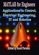MATLAB for Engineers: Applications in Control, EE, IT and Robotics

The book presents several approaches in the key areas of practice for which the MATLAB software package was used. Topics covered include applications for: Motors, Power systems , Robots, Vehicles.

•MATLAB Succinctly (Dmitri Nesteruk)

Learn the essential skills needed to use the flexible MATLAB system. You will be able to apply the highly modular system towards the purposes you need by harnessing the power of its different toolboxes.

•Applications from Engineering with MATLAB Concepts

This book presents a collection of MATLAB-based chapters of various engineering background. Instead of giving exhausting amount of technical details, authors were rather advised to explain relations of their problems to actual MATLAB concepts.

•MATLAB Applications for the Practical Engineer (Kelly Bennett)

This book is a collection of diverse applications of MATLAB. Each chapter presents a novel application and use of MATLAB for a specific result. MATLAB isn't everything to a practicing engineer, its just most everything.

•Numerical Computing with MATLAB (Cleve B. Moler)

This book is a lively textbook for an introductory course in numerical methods, MATLAB, and technical computing. It helps readers learn enough about the mathematical functions in MATLAB to use them correctly, and modify them appropriately, etc.

•Numerical Methods and MATLAB Programming for Engineers

It covers the fundamentals while emphasizing the most essential numerical methods. Readers will enhance their programming skills using MATLAB to implement algorithms, discover how to use MATLAB to solve problems in science and engineering.

•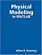Physical Modeling in MATLAB (Allen B. Downey)

Written for beginners, this book provides an introduction to programming in MATLAB and simulation of physical systems. you will learn some programming, some modeling, and some simulation.

•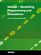MATLAB - Modelling, Programming and Simulations (E. P. Leite)

This is an authoritative guide to generating readable, compact, and verifiably correct MATLAB programs. It is ideal for undergraduate engineering courses in Mechanical, Aeronautical, Civil, and Electrical engineering that require/use MATLAB.

•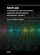MATLAB: Tool for Scientific Computing and Engineering Apps, Vol 1

This is the 1st book in a three-volume series deploying MATLAB-based applications in almost every branch of science. This volume covers topics from different areas of engineering, signal and image processing based on the MATLAB environment.

•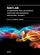MATLAB: Tool for Scientific Computing and Engineering Apps, Vol 2

This is the 2nd book in a three-volume series. This volumehe covers topics electronic engineering and computer science, MATLAB/SIMULINK as a tool for engineering applications, and telecommunication and communication systems.

•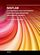MATLAB: Tool for Scientific Computing and Engineering Apps, Vol 3

This is the 3rd book in a three-volume series. This volume consists of mathematical methods in the applied sciences by using MATLAB, MATLAB applications of general interest, and MATLAB for educational purposes.

•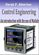Control Engineering: An Introduction with the Use of MATLAB

The book covers the basic aspects of linear single loop feedback control theory. Explanations of the mathematical concepts used in classical control such as root loci, frequency response and stability methods are explained by making use of MATLAB.

•MATLAB - A Ubiquitous Tool for the Practical Engineer (C. Ionescu)

This book is for both students and engineers with wide range of applications in which MATLAB is the working framework, shows that it is a powerful, comprehensive and easy-to-use environment for performing technical computations.

•Engineering Education and Research Using MATLAB (Ali H. Assi)

This book presents research works using MATLAB tools, including techniques for programming and developing GUI, dynamic systems, electric machines, signal and image processing, power electronics, mixed signal circuits, genetic programming, etc.

•Applications of MATLAB in Science and Engineering (Michalowski)

The book illustrates a wide range of areas where MATLAB tools are applied, including mathematics, physics, chemistry, mechanical engineering, biological and medical sciences, communication and control systems, digital signal, etc.

•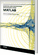Scientific and Engineering Applications Using MATLAB (E. P. Leite)

The purpose of this book is to present 10 scientific and engineering works whose numerical and graphical analysis were all constructed using the power of MATLAB tools.

•Neural Network Toolbox for MATLAB (Howard Demuth, et al)

It provides algorithms, functions, and apps to create, train, visualize, and simulate neural networks. You can perform classification, regression, clustering, dimensionality reduction, time-series forecasting, and dynamic system modeling and control.

•Biological Signal Analysis with MATLAB (R. Palaniappan)

This book will provide the reader with an understanding of biological signals and digital signal analysis techniques such as conditioning, filtering, classification and statistical validation for solving practical biological signal analysis problems using MATLAB.

Book Categories
 :All CategoriesRecent BooksMiscellaneous BooksComputer LanguagesComputer ScienceData Science/DatabasesElectrical EngineeringJava and Java EE (J2EE)Linux and UnixMathematicsMicrosoft and .NETMobile ComputingNetworking and CommunicationsSoftware EngineeringSpecial TopicsWeb Programming
Other Categories